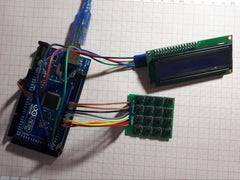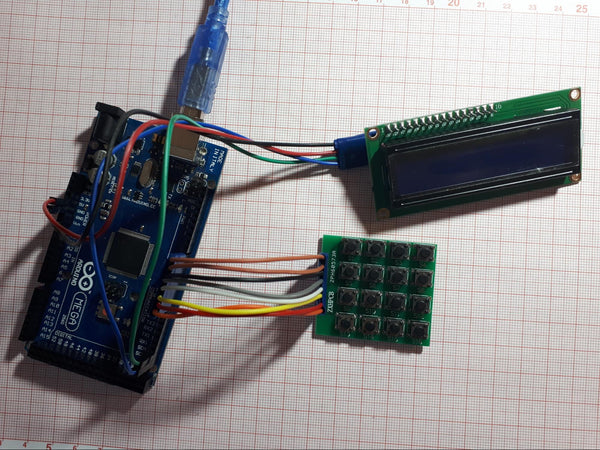# 💻 Caclulator on Arduino, schematics and code

When I learned to do window applications under windows, one of the first was a calculator. Why not try it on Arduino too.

We will need:

• Arduino (Nano, Uno, Mega, ...)
• LCD i2c screen
• Keyboard
• Wires.

The keyboard is used this, but personally, I have another.The keyboard connects to any pins, but it is better to 8 in a row to connect the cable. The LCD screen is connected via an i2c bus.//libraries
#include <LiquidCrystal_I2C.h>
#include <Wire.h>
//cols and rows of keyboard
const byte ROWS = 4;
const byte COLS = 4;
char keys[ROWS][COLS] = {
{'7', '8', '9', 'D'},
{'4', '5', '6', 'C'},
{'1', '2', '3', 'B'},
{'*', '0', '#', 'A'}
};
//keyboard pins
byte rowPins[ROWS] = { 0, 1, 2, 3 };
byte colPins[COLS] = { 4, 5, 6, 7 };
LiquidCrystal_I2C lcd(0x26, 16, 2);
long Num1, Num2, Number;
char key, action;
boolean result = false;
void setup() {
//turning on lcd
lcd.init();
lcd.backlight();
Serial.begin(9600);
}
void loop() {
//get key
key = kpd.getKey(); //storing pressed key value in a char
if (key != NO_KEY) DetectButtons();
if (result == true) CalculateResult();
//display result
DisplayResult();
}
//detect buttons
void DetectButtons()
{
lcd.clear();
if (key == '*')
{
Serial.println ("Button Cancel");
Number = Num1 = Num2 = 0;
result = false;
}
if (key == '1')
{ Serial.println ("Button 1");
if (Number == 0)
Number = 1;
else
Number = (Number * 10) + 1;
}
if (key == '4')
{ Serial.println ("Button 4");
if (Number == 0)
Number = 4;
else
Number = (Number * 10) + 4;
}
if (key == '7')
{ Serial.println ("Button 7");
if (Number == 0)
Number = 7;
else
Number = (Number * 10) + 7;
}

if (key == '0')
{ Serial.println ("Button 0");
if (Number == 0)
Number = 0;
else
Number = (Number * 10) + 0;
}
if (key == '2')
{ Serial.println ("Button 2");
if (Number == 0)
Number = 2;
else
Number = (Number * 10) + 2;
}
if (key == '5')
{ Serial.println ("Button 5");
if (Number == 0)
Number = 5;
else
Number = (Number * 10) + 5;
}
if (key == '8')
{ Serial.println ("Button 8");
if (Number == 0)
Number = 8;
else
Number = (Number * 10) + 8;
}

if (key == '#')
{ Serial.println ("Button Equal");
Num2 = Number;
result = true;
}
if (key == '3')
{ Serial.println ("Button 3");
if (Number == 0)
Number = 3;
else
Number = (Number * 10) + 3;
}
if (key == '6')
{ Serial.println ("Button 6");
if (Number == 0)
Number = 6;
else
Number = (Number * 10) + 6;
}
if (key == '9')
{ Serial.println ("Button 9");
if (Number == 0)
Number = 9;
else
Number = (Number * 10) + 9;
}
if (key == 'A' || key == 'B' || key == 'C' || key == 'D')
{
Num1 = Number;
Number = 0;
if (key == 'A')
{
Serial.println ("+");
action = '+';
}
if (key == 'B')
{
Serial.println ("-");
action = '-';
}
if (key == 'C')
{
Serial.println ("*");
action = '*';
}
if (key == 'D')
{
Serial.println ("/");
action = '/';
}
delay(100);
}
}
//calculate result
void CalculateResult()
{
if (action == '+')
Number = Num1 + Num2;
if (action == '-')
Number = Num1 - Num2;
if (action == '*')
Number = Num1 * Num2;
if (action == '/' && Num2 !=0)
Number = Num1 / Num2;
}
//display result
void DisplayResult()
{
lcd.setCursor(0, 0);
lcd.print(Num1); lcd.print(action); lcd.print(Num2);
if (result == true)
{
lcd.print(" =");
lcd.print(Number);
}
lcd.setCursor(0, 1);
lcd.print(Number);
}
Turn on the calculator and enjoy his work.
In the bottom line, we still have a sketch calculator on an Arduino with a 16 * 2 screen and any 4 * 4 keyboard. Forward to new heights!